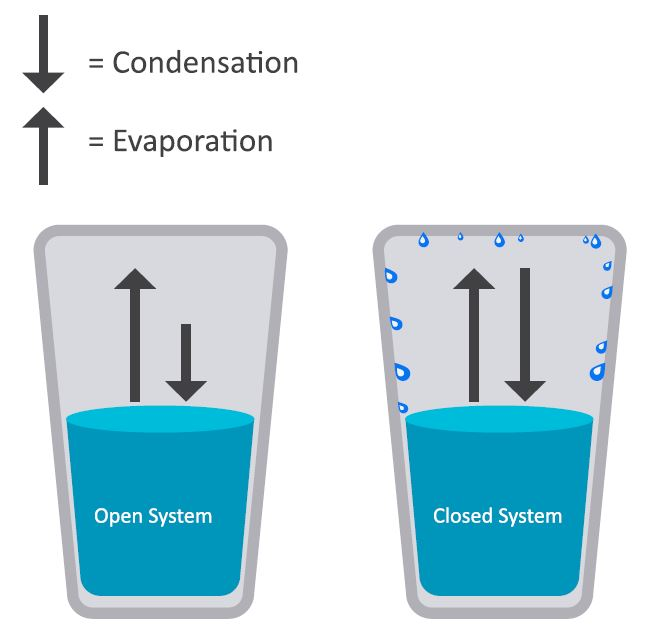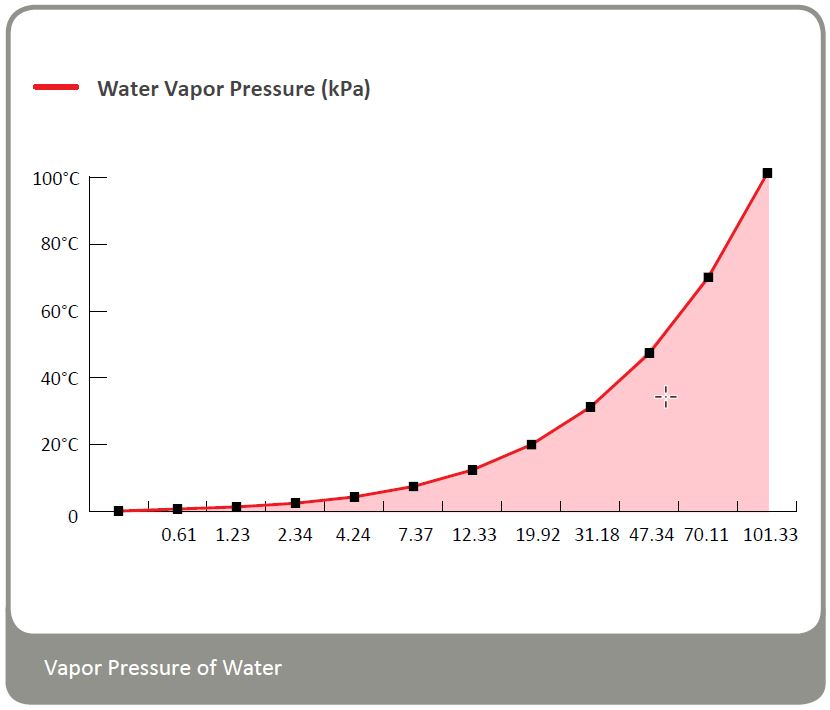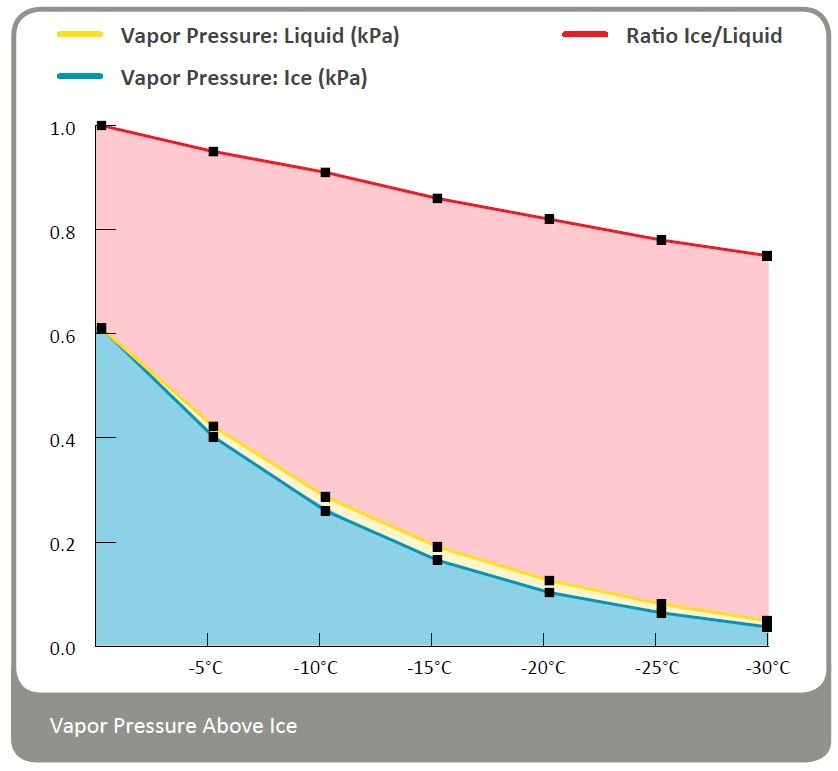# Humidity Academy Theory 3 – Humidity and Vapor Pressure

Water vapor is one of several gases that makes up air. For example if the total pressure of a system such as air at sea level is 101.3 kPa (or 29.9 inches of mercury), and that air is made up of Nitrogen, Oxygen, water vapor and other trace gases, each of those gases contributes to the total pressure of 101.3 kPa. The portion that is water vapor is called the partial pressure of water vapor. The partial pressure of water vapor is a key metric found as a component in the formulas that define all other humidity parameters.

## Effect of a Change in Pressure

Dalton’s law:

According to Dalton’s law, the total pressure of a gas mixture is equal to the sum of the partial pressures of its components. This means that the partial pressure of a component is equal to the product of the total pressure times the mole fraction of the component.

Therefore, a change in the total pressure of a gas mixture, at constant composition, results in the same change in the partial pressure of each component. For instance, doubling the total pressure of a gas mixture results in doubling the partial pressure of each component. As the total pressure increases, the partial pressure of water vapor increases proportionately. This is an important fact to understand, as you will see when we define relative humidity and dew point temperature. An increase in the pressure of a closed system will increase the relative humidity and raise the dew point temperature until saturation is achieved.

## Vapor Pressure Above a LiquidBecause molecules in a liquid are closer to one another than they are in a gas, intermolecular forces are stronger than in a gas. For a liquid to vaporize, the intermolecular forces have to be overcome by the kinetic energy of the molecules. If a liquid is placed in a closed container, the particles entering the vapor phase cannot escape. In their random motion, particles strike the liquid and are recaptured by intermolecular forces. Thus, two processes occur simultaneously: evaporation and condensation. The rate of evaporation increases as temperature increases. This is because an increase in temperature corresponds to an increase in the kinetic energy of molecules. At the same time, the rate of condensation increases as the number of particles in the vapor phase increases: more molecules strike the surface of the liquid.
When these two processes become equal, the number of particles and, therefore, the pressure in the vapor phase, becomes stabilized.
The value of the equilibrium vapor pressure depends on the attractive forces between particles of the liquid and on the temperature of the liquid. Vapor pressure above a liquid increases with increasing temperature.

## Saturation Water Vapour PressureWater vapor pressure saturates strongly with increasing temperature.

## Vapor Pressure Above IceWhen water freezes, the molecules assume a structure that permits the maximum number of hydrogen-bonding interactions between molecules. Because this structure has large hexagonal holes, ice is more open and less dense than liquid water. As hydrogen bonding is stronger in ice than in liquid water, the inter-molecular attraction forces are the strongest in ice. That’s why vapor pressure above ice is less than the vapor pressure above liquid water.